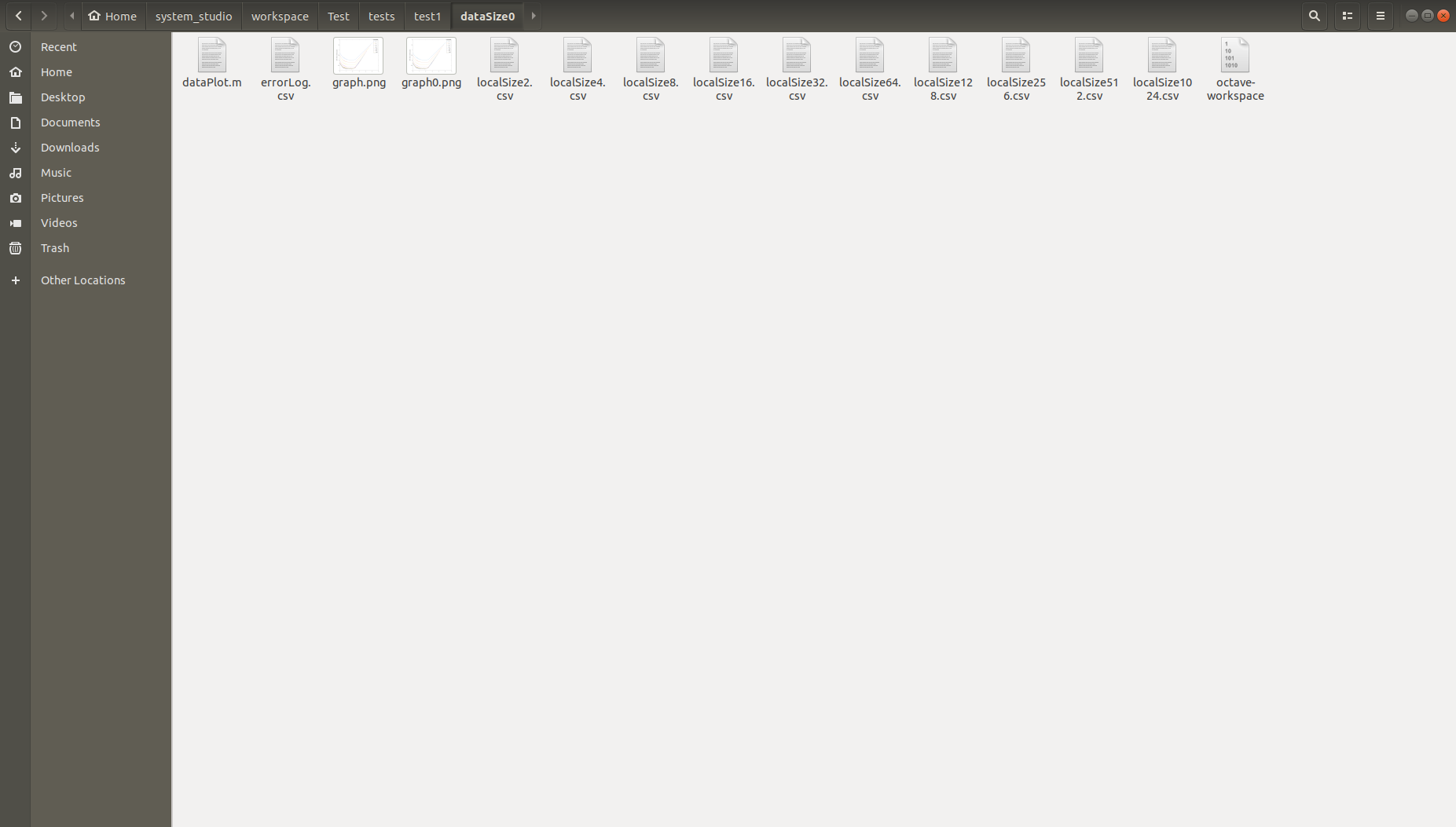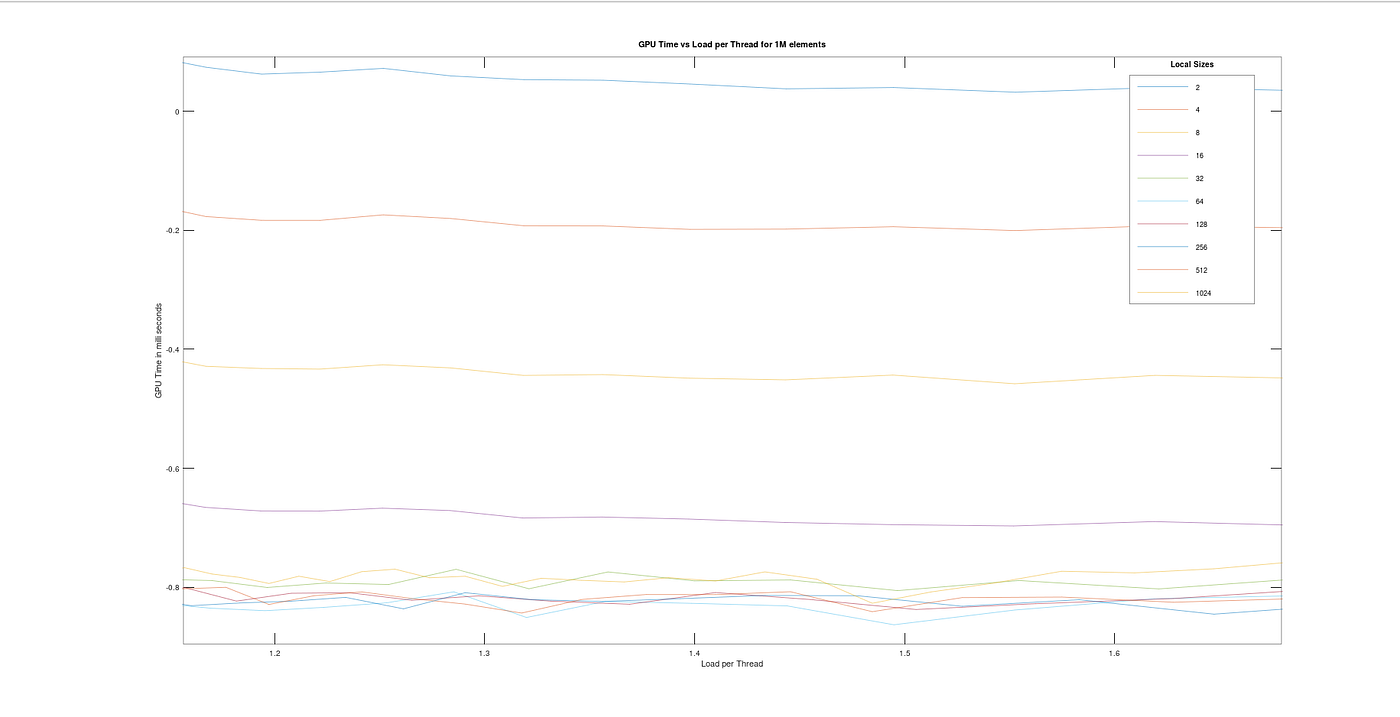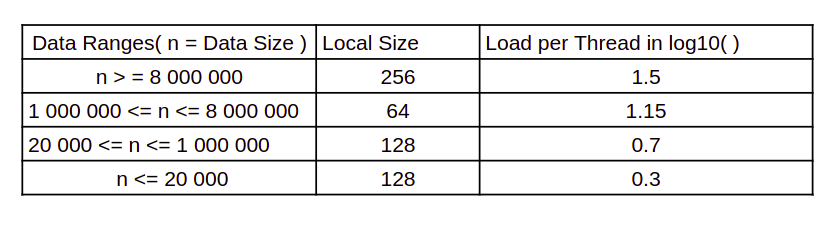# Super Fast Addition in GPU — II

Last time we discussed about how much impact a GPU can have upon a program which includes large number of processing data compared to performance of a CPU ( the article can be read from here ). The question is ‘will that fast implementation on GPU be the fastest program out of many possible implementations?’ . In this article I would like to answer this particular question with a precise experimental methodology.

## Step 1: Identifying Suitable Parameters for the Test

When we measure the performance of the reduce algorithm, following parameters can be identified as decisive factors of it.

1. Size of input data
2. Local size of the Kernel
3. Total work done by a single work item
4. Execution time inside GPU

Since execution time in GPU is directly not in our control, we keep it as a dependent variable. The other factors are highly correlated with each other. Observing a 3D space can be a much more exhausting task; therefore I decided to continue the experiment with 2D graphs. For a single data size, several graphs are plotted for all the local sizes with work done by single work item as independent variable. For different data sizes the above procedure was continued.

## Step 2: Developing the Test

It is quite obvious that we cannot tune those parameters for each and every data size. Therefore in this test I defined several data sizes for different ranges in such a way that every range includes 4 data sizes.

• 10³ — 10⁴
• 10⁴ — 10⁵
• 10⁵ — 10⁶
• 10⁶ — 8 x 10⁶

Let’s take there are n data sizes d0, d1, d2, …….. dn
and m local sizes L1, L2, L3, ……Lm and k work groups G1, G2, G3, …… Gk

In order to obtain some good understanding about how work items work with different loads, instead of choosing an axis as Local Size; load per thread was used. According to my algorithm `loadPerThread = DATA / (2 * LOCALSIZE * NUMBER_OF_WORKGROUPS)` . Since the minimum number of data per work group should be 2, there was a maximum number of work groups. `maxWorkGroups = DATA / (2 * LOCALSIZE)` . Therefore different amounts of data points were obtained for different local sizes even in the same data size.

For each data size and local sizes, I obtained GPU time and wrote them into csv files. All the results in GPU were compared with relevant CPU result to ensure the accuracy of the answer.Different data sizes and csv files for each local size

## Step 3: Plotting graphs for collected data

Octave, which is a software developed for numerical calculations in high level language was used in plotting the graphs. This is very similar to Mat Lab but completely free for use. Those graphs looked like follows.

A simple observation in the above graph reveals that every local size reflects a graph with a minima of time at a certain point of load per thread. Out of them there is one graph which has the minimum out of all minimas which is exactly what we are interested in. A zoomed version of those graphs might help to figure this out.Zoomed version of graphs obtained for 10⁶ data size

## Step 4: Determining optimal parameter values

As mentioned above we have now selected graphs with minimum execution time occurrence in each data size. Depending on the similarity of their local sizes, data ranges have been identified. To obtain the optimum load per thread, those graphs in the same range have been plotted in the same figure. Followings are the optimum parameter values obtained from the experiment.Lookup table for the final reduce host code

The above lookup table has been included in the final implementation of reduce host code which is displayed below.

You can access the complete project I have developed from here.

--

--